# Chapter 1: Probability ( basic review of sets)

#### Definition :

A set is a collection of mathematical or every kind of elements  e.g   A = {1,2,3,4,6,Δ,a} .1,2,3,4,6,Δ,a  are called elements of the set A and the size of elements is the absolute value of A = !A! (that is the absolute avalue of  total number of elements) .In this case the size of A is 7

Operation on sets:

There is a kind of mathematical rules of sets:  ∩= Intersection,  U= union eg If A and B are sets  AUB = is the set of all X such that X is an element of A or X is an element of B  A∩B= is the set of all X such that X belongs to A and X belongs to B. Sometimes we can do a kind of venn diagrams to illustrate sets :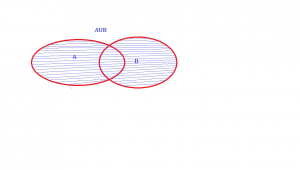Sometimes all sets belong to a universal set eg the universal set U then A complement is a set of X belonging to U such that X dose not belong to A

A U (B ∩ C) = (A U B) ∩(A U C)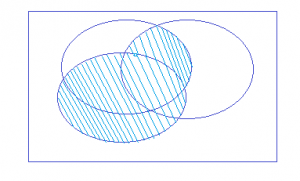That means every thing in A plus every thing in the intersection of B and C

AUBUC = ¦A¦+¦B¦+¦C¦—¦( A∩B)¦—¦A∩C¦—¦B∩C¦+¦A∩ B∩ C¦

Inclusion/Exclusion Inclusion/ Exclusion for n sets!

Ex. Uni  foot team has 11 students taking chemistry (C) ,12 students taking mathematics (M),12 students taking physics (P). If 7 take C and P, If 4 take M and P, If 5 take C and M, If 3 take all three, If 3 take none of these.Haw many are in the team?

so without any formulas we have 25(Number) in the union (total =25)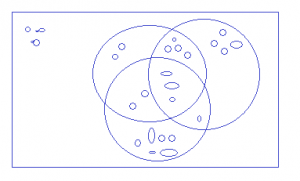Partition of a set A:

the idea of partition is to subdivide a set in pieces

Def: a partition of the set A is a set of subsets

If C is a subset of B that means if X belongs to C then X belongs to B. the addition of subsets equals the original set

There are conditions to that : all the subsets of A must be  disjoint. so A1+A2+A3+…………………………..Ak= A

Ex :

Let’s flip a coin 3 times then the possible outcomes are 8 possibilities (TTT, TTH, THT, THH, HTT, HTH, HHT,HHH) .2×2×2 ( every time there are 2 possibilities) now lets consider a partition :

A0 :{TTT}      A1:{TTH,THT,HTT}    A2:{THH,HTH, HHT}      A3 :{HHH}

AK : set of outcomes with K heads , this is a partition of big set A

Functions:

Suppose that A and B are sets and f(A) that means inputs on element A are the outputs on element B associated with it .

We can define im ( f)={ b belonging to B } For each b we can define the inverse image (b)

# Chapter 2: basic counting and probability

Basic counting and probability

Let’s count the number of words of length  K with n letters  ( repetition is  authorized): So  we have   A ,  B , B ,A ,  C ,   (K = 5    n= 3 )   generally   we  can wright (n exponant k ) words.

Now let’s count words from alphabet of k letters with repetion not allowed: K= 5  this kind of counting is called permutation of k objects :

A  B  C   D   E ( in this kind of counting each letter can be used only once So we will have 5 choices for the first letter , 4 for the second ,3 for the third ,2 for the forth , and 1 for the fifth .

K (K-1)(k-2)(k-3)(k-4) 1 =K!

Now let’s count words of length K from alphabet of n letters :N choices for the first letter,(N-1) choices for the second letter,(N-2 )choices for the third letter……(N-k)+1 choices for k th letter .That means n!/(n-k)! Words and without permutation we must divide by K!   so thefinal formula will be :   n!/(n-k)!k!   (that is the binomial coefficient)So for example for(3 exponant 5 )  we will have 5!/(5-3)!3! = 10   eg : from  A,B,C,D,E  we can have 10 possibilities:

{A,B,C}    {A,B,D}    {A,B,E}   {A,C,D}    {A,C,E}   { A,D,E}    {B,C,D}    {B,C,E}    {B,D,E}   {C,D,E}

BASIC DEFINITION OF PROBABILITY

Suppose a certain outcome o from N ways out of T possibilities. this is between 0 and 1    0 <  p <1

Ex:  Rolling a dice the Probability of getting a 3= 1/6  and  in a class of 28 peoples, 4 have red hair. Probability of red hair = 4/28 = 1/7

Ex: Probability of getting 3 successive Heads if you flip a coin 3 times = 1/2 x 1/2 x 1/2 = 1/8

Ex: Rolling tow dices the probability of getting 8 : well in this case the space of

Possibility is( total number is 36 and)  and outcomes giving 8 are 7 so the probability is  = P = 7/36

RULES FOR PROBABILIOTY

Negation Rule: if the probability of an outcome o is P then the probability of O not occurring is (1-P).

Ex: Probability of rolling a 3 is 1/6 so that the probability of not rolling a 3 = 5/6

Multiplication Rule: if O1 and O2 are independent outcomes that means knowing that one does not influence the other. Then the probability of (O1 and O2) occurring together is the Prob(O1) x Prob(O2)

Ex: Wat´s the probability of drowing a red face card (Jack,Queen,King).

Red cards= hearts and diamonds . Wat`s the probability of getting a red card =2/4 and the Probability of getting a face card = 3/13 so the probability of drowing a red face card is 2/4 x 3/13 = 6/52 = 3/2

Addition Rule: if an outcome O can occur in two or more distinct possibles ways, then the probability of O is the  some   of the probabilities of each of individual ways of getting O.

Ex: Probability of getting  2 (3) if we roll a dice 5 times:Probability of getting a 3 in exactly first and forth rolles  is  the same  and not getting 3 in the second rol = 1-4/6 Now the probability of any of two positions   Then final probability =0,161

POCKER CALCULATION

What is the probability of getting at least one pair from of 5 card hand (out of 52) .1-P=probability of getting all cards from different values 1   so   P=1-0,4929=1/2

Common birthdays: What´s the probability of ten people in the room at least two share birthday.Let´s find that  1-P=all ten have distinct birthday1-P=1    so P=1-0,883=0,117   and With 23 people P

ThisisbetweenProbabilityofgettingEx:

# Chapter 3a: the motion- the position

The first formalism that has to be known in physics is the formalism of the motion. A formalism is associated with a certain rigorous mathematical method, defining symbols and rules that are commonly accepted, in the goal that everybody understands immediately the discussed matter. We will not be interested in the prediction of the motion nor its cause, but in its description alone.

In this section, we consider that every object can be considered as a dot without volume.

The position

The motionis the history of the position of an object, the succession of the positions of this object over the time. To define the position of the object, we need a reference system in which we can give the position: coordinates. Several systems of reference exist and there are several ways to calculate the coordinates of objects. All of them are corrects but some are more convenient than the others. During a trip, you won’t give your position with regard to the sun, the same is true in physics.

Several coordinates are generally necessary to determine the exact position of one object. If you say that you are 10km from Paris, you give an information on your position but we are lacking at least one coordinate to determine your position. Usually you need one coordinate by dimension of the system.

One dimension

We choose one origin to the coordinate system, the zero point. It is convenient to choose the initial position of the object A as the origin but it is not mandatory. Next we choose a direction that will be the positive positions. In the opposite direction we have the negative positions. Still for convenience, the positive positions are placed in the direction we guess the object will move towards. Imagine that the object moves towards another object B placed at a distance d. The position vector indicates the distance between an object and the origin, and points towards the object with an arrow. The symbol for vectors is topped by an arrow pointing to the right. The position vector for B is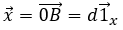Where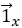is the unit vector in the direction x (the single direction in this problem).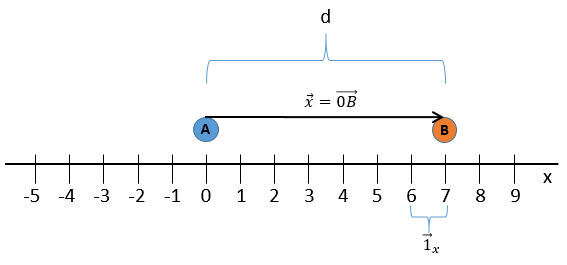Two dimensions

The second coordinate is usually orthogonal, perpendicular to the first coordinate to avoid a maximum of angle problems and to benefit the simplicity of the calculation for right triangles. Each coordinate has a direction.

The position vector is now defined by two components from which we can calculate its length if desired.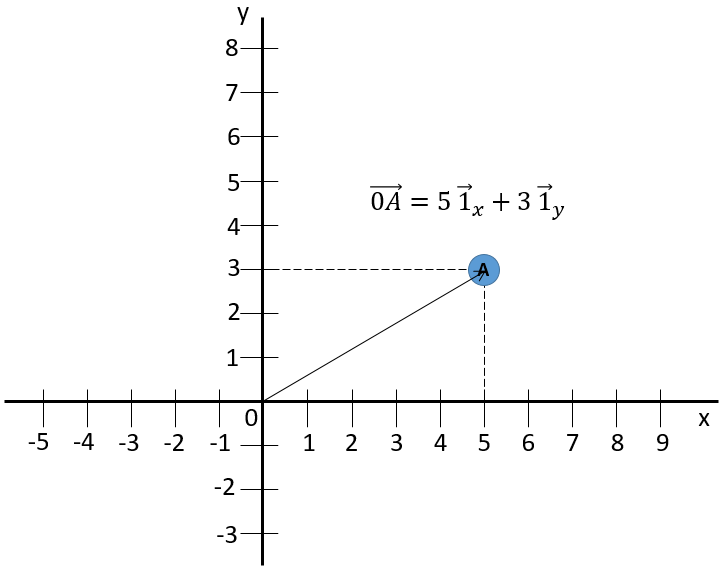The addition sign between the two unit vectors is seen as “followed by” and not like the addition of two usual numbers. Another way to write coordinates is to put them in brackets. If we do this, then we don’t write the unit vectors.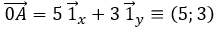This system of reference, the Cartesian system, is not the single one that can be used at two dimensions to determine the position of an object. We can also position the object from its distance r to the origin point and an angle θ from one axis. This reference system is called the polar system.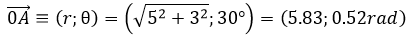It is possible to determine the relation between the coordinates X;Y in the Cartesian system and r;θ in the polar system using the relation defining the cosine, the sinus and the tangent: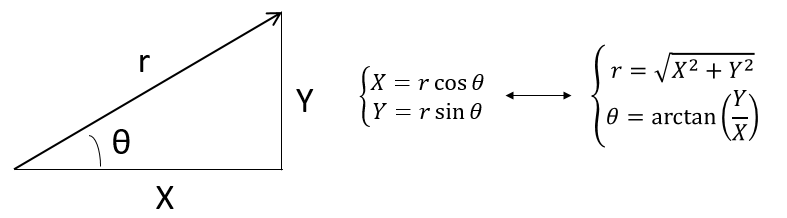The unity vector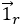can thus be calculated.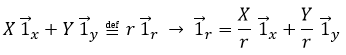or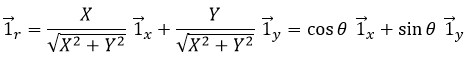We can also define the unit vector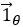: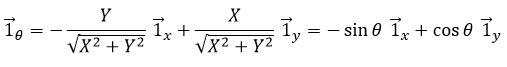Both polar unity vectors depend thus upon θ that should thus be chosen conscientiously.

Three dimensions

A third coordinate is added in the reference systems. In the Cartesian system, we add a coordinate that is orthogonal to the two previous ones.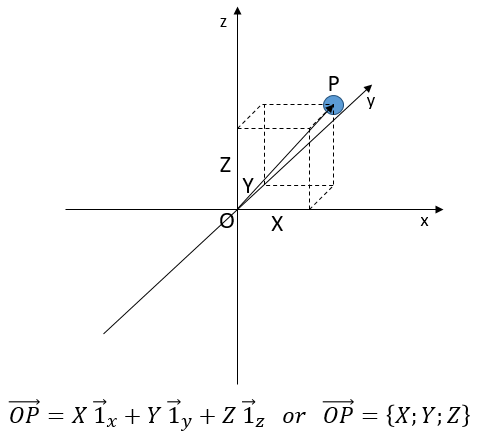In the polar system, we need a second angle to determine the position of an object. We take the first from the axis x in the xy plane and the second angle is taken from the z axis in the zr plane of the object.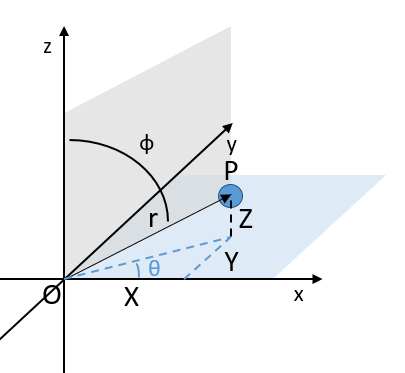Again, the Cartesian system can be associated to the polar system.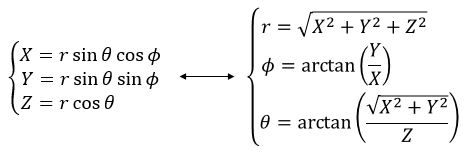The unit vectors are defined as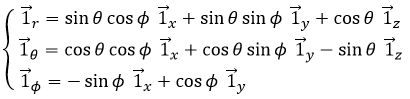# Total Probability, Bayes rule, Tree diagrams

Probability spaces: Sample space S, Probability measure PΣ p(si) = 1        si<1   Event E is a subset of S,  so:  p(E) = Σ (ps)             S<E

Definition:  events A  and  B are  independent so  P(A ∩ B)=P(A) P(B), We  can extend  this to more than 2 subsets: A,B,C S , A,B,C=events, =are mutually independent that means A and B are independents, A and C are independents and B and C are independents:

P(A∩B)=P(A) P(B)      P(A∩C)=P(A) P(C)          P(B∩C)=P(B) P(C)              P(A∩B∩C)=P(A) P(B) P(C)

For more than 3 sets ,We can generalize this : p {A1∩A2∩A3∩A4∩A5∩…….}=  P(A1) P(A2) P(A3)P(A4)p(A5) ………

Conditional probability :P(A\B) =  p(A∩B)/p(B),      Note: if A and B are independent then P(A\B) =P(A) because  P(A)p(B)/p(B)= p(A)

Total Probability ruleSuppose {A1,A2,A3,A4,…….An ) is a partition of S, that means these are subsets which together they form all of S (without overlap).Suppose B is an event in S. Then the probability of B equals the sum:P(B) =Σ p(B\Ai) p(Ai)

Proof: B=(B∩A1 )U (B∩A2 )…….. U(B∩An) .This is a disjoint union so:

P(B)=P(B∩A1 )+P(B∩A2 )+………..+P(B∩An )= P  P(B\A1 )PA1 +P(B\A2)PA2 …………P(B\An)  P An

Ex: does it rain more on week-ends? After a year of observation, we find that the probability of rain on a work day (W) is represented by 0,2.

Let’s also suppose that the probability of rain on a week-end day (H) is 0,3. With this we can probably say that it is more likely to rain on a day of weekend than the ordinary day.

Question: What is the overall probability of rain on a random day?

This question is usefull to introduce the tree diagram

What is the probability of rain? Total Probability:P(R)=P(R\W)  P(W)+P(R\H) P(H)  =0,2 X5/7 +0,3X2/7 =0,2286

Bayes Rule

Recall that P(A/B)  is not symmetric so  P(A/B) ≠ P(B/A)  ,  Bayes rule:

If 〈 A1,A2,A3,A4,A5……An〉 is a partition of S and B ≤ S (an event), then for any j,  the P(Aj/B)= P(B/Aj) P(Aj)

Proof:        P(Aj/B)=  P(Aj∩B)/p(B)

p(B/Aj)=  P(Aj∩B)/p(Aj)

So :   P( Aj/B) = P(B/Aj)p(Aj)/p(B)

and in general : P(Aj/B) = P(B/Aj)P(Aj)/ ∑P(B/Ai)P(Ai)

Let’s look to the situation of raining day. Suppose on a certain day it rains and we are intersected to know, what’s the probability that happens to be a week-end day ? Well according to the Bayes Rule

P (H/R)= P(R/H)P(H)/P(R)  = p(R/H)p(H)/p(R/W)p(W)+p(R/H)p(H)

= 0,3×2/7 divided by :0,2×5/7+0,3×2/7

Medical ex:

If you take a medical test is + but tests are not infaillable.A random person has a disease (D) or not ( ),Let’s suppose that we know that 3% of the population has this disease.The test is differing on actually if you have the disease. The test for D is 99%  sure of returning (+) if the persons has the disease and 98% sure of correctly returning (-) if they do not have it.Given Y what is the probability of D?

P

# Chapter 5 : exercices and exam questions (probability)

Problem 1:

A marketing survey indicates that 60% of the population owns an automobile, 30% owns a house, and 20% owns both an automobile and house. Calculate the probability that a person chosen at random owns an automobile or a house, but not both.

(A) 0.4               (B) 0.5                     (C) 0.6               (D) 07              (E) 09

Problem 2:

The probability that a visit to a primary care physician’s (PCP) office results in neither lab work nor referral to a specialist is 35% of those coming to a PCP’s office, 30% are referred to specialists and 40% require lab work. Determine the probability that a visit to a PCP’s office results in both lab work and referral to a specialist.

(A) 0.05               (B) 0.12                     (C) 0.18               (D) 0.25              (E) 0.35

Problem 3:

You are given P (A∪B) =0.7 and P (A∪B’) =0.9 Determine P (A).

(A)   0.2               (B) 0.3                     (C) 0.4               (D) 0.6              (E) 0.8

Problem 4:

Workplace accidents are categorized into three groups: minor, moderate, severe. The probability that a given accident is minor is 0.5, that it is moderate is 0.4, and that it is server is 0.1. Two accidents occur independently in one month. Calculate the probability that neither accident is severe nor at most one is moderate.

(A)   0.25               (B) 0.40                     (C) 0.45               (D) 0.56              (E) 0.65

Problem 5:

An actuary studying the insurance preferences of automobile owners makes the following conclusions:

-An automobile owner is twice as likely to purchase collision coverage as disability coverage.

-The event that an automobile owner purchases collision coverage is independent of the event that he or she purchases disability coverage.

-The probability that an automobile owner purchases both collision and disability coverages is 0.15.

What is the probability that an automobile owner purchases neither collision nor disability coverage?

(A)   0.18              (B) 0.33                     (C) 0.48               (D) 0.67              (E) 0.82

Problem 6:

An insurance company estimates that 40% of policyholders who have only an auto policy will renew next year and 60% of policyholders who have only a homeowner’s policy will renew next year. The company estimates that 80% of policyholders who have both an auto and a homeowner’s policy will renew at least one of those policies next year. Company records show that 65% of policyholders have an auto policy, 50% of policyholders have a homeowner’s policy, and 15% of policyholders have both an auto and a homeowner’s policy. Using the company’s estimates, calculate the percentage of policyholders that will renew at least one policy next year.

(A)   20              (B) 29                     (C) 41               (D) 53               (E) 70

Problem 7:

A survey of a group’s viewing habits over that last year revealed the following information:

-28% watched gymnastics.

-29% watched baseball.

-19% watched soccer.

-14% watched gymnastics and baseball.

-12% watched baseball and soccer.

-10% watched gymnastics and soccer.

-8% watched all three sports.

Calculate the percentage of the group that watched none of the three sports during the last year.

(A)   24              (B) 36                     (C) 41               (D) 52               (E) 60

Problem 8:

An insurance company pays hospital claims. The number of claims that include emergency room or operating room charges is 85% of the total number of claims. The number of claims that do not include emergency room charges is independent of the occurrence of operating room charges on hospital claims. Calculate the probability that a claim submitted to the insurance company includes operating room charges.

(A)   0.10              (B) 0.20                     (C) 0.25               (D) 0.40               (E) 0.80

Problem 9:

A blood test indicates the presence of a particular of a disease 95% of the time when the disease is actually present. The same test indicates the presence of the disease 0.5% of the time when the disease is not present. One percent of the population actually has the diseases. Calculate the probability that a person has the disease given that the test indicates the presence of the disease.

(A)   0.324            (B) 0.657              (C) 0.945                (D) 0.950                 (E) 0.995

Problem 10:

Ten present of a company’s life insurance policyholders are smokers. The rest are non-smokers. For each non-smoker’s the probability of dying during the year is 0.01. For each smoker, the probability of dying during the year is 0.05. Given that a policyholder has died, what is the probability that the policyholder was a smoker?

(A)   0.324            (B) 0.657            (C) 0.945                (D) 0.950                 (E) 0.995

Problem 11:

An auto insurance company insures divers of all ages. An actuary compiled the following statistics on the company’s insured drivers:

 Age of driver Probability of accident Portion of company’s insured drivers 16-20 0.06 0.08 21-30 0.03 0.15 31-65 0.02 0.49 66-99 0.04 0.28

A randomly selected driver that the company insures ha an accident. Calculate the probability that the driver was age 16-20.

(A)   0.13            (B) 0.16                     (C) 0.19                (D) 0.23                 (E) 0.40

Problem 12:

An actuary studied the likelihood that different types of drivers would be involved in at least one collision during any one-year period. The results of the study are presented below.

 Type of driver Percentage of all drivers Probability of at least one collision Teen 8% 0.15 Young adult 16% 0.08 Midlife 45% 0.04 Senior 31% 0.05

Given that a driver has been involved in at least one collision in the past year, what is the probability that the driver is a young adult driver?

(A)   0.06            (B) 0.16                   (C) 0.19                (D) 0.22                 (E) 0.25

Problem 13:

A health study tracked a group of persons for five years. At the beginning of the study, 20% were classified as heavy smokers, 30% as light smokers, and 50% as non-smokers. Results of the study showed that light smokers were twice as likely as non-smokers to die during the five-year study, but only half as likely as heavy smokers. A randomly selected participant from the study died over the five-year period. Calculate the probability that the participant was a heavy smoker.

(A)   0.20            (B) 0.25                    (C) 0.35                (D) 0.42                 (E) 0.57

Problem 14:

The probability that a randomly chosen male has a circulation problem is 0.25. Males who have a circulation problem are twice as likely to be smokers as those who do not have circulation problem. What is the conditional probability that a male has a circulation problem, given that he is a smoker?

(A)   1/4            (B) 1/3                    (C) 2/5                (D) 1/2                 (E) 2/3

Problem 15:

A doctor is studying the relationship between blood pressure and heartbeat abnormalities in her patients. She tests a random sample of her patients and notes their blood pressures (high, low, or normal) and their heartbeats (regular or irregular). She finds that:

-14% have high blood pressure

-22% have low blood pressure

-15% have an irregular heartbeat

-Of those with an irregular heartbeat , one-third have high blood pressure

-Of those with normal blood pressure, one-eighth have an irregular heartbeat

What portion of the patients selected have a regular heartbeat and low blood pressure?

(A)   2%            (B) 5%                    (C) 8%                (D) 9%                 (E) 20%

Problem 16:

An urn contains 10 balls, 4 red and 6 blue. A second urn contains 16 red balls and an unknown number of blue balls. A single ball is drawn from each urn. The probability that both balls are of the same color is 0.44. Calculate the number of blue balls in the second urn.

(A)   4                 (B) 20                    (C) 24                (D) 44              (E) 64

Problem 17:

A public health researcher examines the medical records of a group of 937 men who died in 1999 and discovers that 210 of the men died from causes related to heart disease. Moreover, 312 of the 937 men had at least one parent who suffered from heart disease, and, of these 312 men, 102 died from causes related to heart disease. Determine the probability that a man randomly selected from this group died of causes related to heart disease, given that neither of his parents suffered from heart disease.

(A)   0.115               (B) 0.173                (C) 0.224             (D) 0.327              (E) 0.514

Problem 18:

Among a large group of patients recovering from shoulder injuries, it is found that 22% visit both a physical therapist and a chiropractor, whereas 12% visit neither of these. The probability that a patient visits a chiropractor exceeds by 0.14 the probability that a patient visits a physical therapist. Determined the probability that a randomly chosen member of this group visits a physical therapist.

(A)   0.26               (B) 0.38                    (C) 0.40             (D) 0.48               (E) 0.62

Problem 19:

An insurer offers a health plan to the employees of a large company. As part of this plan, the individual employees may choose exactly two of the supplementary coverages A, B, and C, or they may choose no supplementary coverage. The proportions of the company’s employees that choose coverages A,B, and C are 1/4, 1/3, and 5/12, respectively. Determined the probability that a randomly chosen employee will choose no supplementary coverage.

(A)   0               (B)  47/144                   (C) 1/2           (D) 97/144               (E)7/9

Problem 20:

An insurance company issues life insurance policies in three separate categories: standard, preferred, and ultra-preferred. Of the company’s policyholders, 50% are standard, 40% are preferred, and 10% are ultra-preferred. Each standard policyholder has probability 0.010 of dying in the next year, each preferred policyholder has probability 0.005 of dying in the next year, and each ultra-preferred policyholder has probability 0.001 of dying in the next year. A policyholder dies in the next year. What is the probability that the deceased policyholder was ultra-preferred?

(A)   0.0001        (B) 0.0010               (C) 0.0071           (D) 0.0141          (E) 0.2817

Problem 21:

Upon arrival at a hospital’s emergency room, patients are categorized according to their condition as critical, serious, or stable. In the past year:

-10% of the emergency room patients were critical;

-30% of the emergency room patients were serious;

-The rest of the emergency room patients were stable;

-40% of the critical patients died;

-10% of the serious patients died; and

-1% of the stable patients died.

Given that a patient survived, what is the probability that the patient was categorized as serious upon arrival?

(A)   0.06               (B) 0.29                 (C) 0.30             (D) 0.39               (E) 0.64

Problem 22:

A large pool of adults earing their first driver’s license includes 50% low-risk drivers, 30% moderate-risk drivers, and 20% high-risk drivers. Because these drivers have no prior driving record, an insurance company considers each driver to be randomly selected from the pool. This month, the insurance company writes 4 new policies for adults earning their first driver’s license. What is the probability that these 4 will contain at least two more high-risk drivers than low-risk drivers?

(A)   0.006               (B) 0.012                (C) 0.018             (D) 0.049              (E) 0.073

Problem 23:

A study of automobile accidents produced the following data:

 Model year Proportion ofall vehicles Probability of involvement in an accident 1997 0.16 0.05 1998 0.18 0.02 1999 0.20 0.03 Other 0.46 0.04

An automobile from one of the model year 1997, 1998, and 1999 was involved in an accident.

Determine the probability that the model year of this automobile is 1997.

(A)   0.22               (B) 0.30                (C) 0.33             (D) 0.45              (E) 0.50

Problem 24:

An insurance company examines its pool of auto insurance customers and gathers the following information:

-All customers insure at least one car.

-70% of the customers insure more than one car.

-20% of the customers insure a sports car.

-Of those customers who insure more than one car, 15% insure a sports car.

Calculate the probability that a randomly selected customer insures exactly one car and that car is not a sports car.

(A)   0.13               (B) 0.21                (C) 0.24             (D) 0.25              (E) 0.30

Problem 25:

An auto insurance company has 10,000 policyholders. Each policyholder is classified as

-Young or old;

-Male or female; and

-Married or single.

Of these policyholders, 3000 are young, 4600 are male, and 7000 are married. The policyholders can also be classified as 1320 young males, 3010 married males, and 1400 young married persons. Finally, 600 of the policyholders are young married males. How many of the company’s policyholders are young, female, and single?

(A)   0.13               (B) 0.21                (C) 0.24             (D) 0.25              (E) 0.30

Problem 26:

A dental insurance policy cover three procedures: orthodontics, fillings, and extractions. During the life of the policy, the probability that the policyholder needs

-Orthodontic work is ½;

-Orthodontic work or a filling is 2/3;

-Orthodontic work or an extraction is 3/4;

-A filling and an extraction is 1/8.

the need for orthodontic work is independent of the need for a filling and is independent of the need for an extraction. Calculate the probability that the policyholder will need a filling or an extraction during the life of the policy.

(A)   7/24               (B) 3/8                (C) 2/3             (D) 17/24              (E) 5/6

Problem 27:

An actuary is studying the prevalence of three health risk factors, denoted by A, B, and C, within a population of women. For each of the three factors, the probability is 0.1 that a woman in the population has only this risk factor (and no others). For any two of the three factors, the probability is 0.12 that she has exactly these two risk factors (but not the other). The probability that a woman has all three risk factors, given that she has A and B, is 1/3. What is the probability that a woman has none of the three risk factors, given that she does not have risk factor A?

(A)   0.280             (B) 0.311                (C) 0.467              (D) 0.484              (E) 0.700

Problem 28:

An insurance company designates 10% of its customers as high risk and 90% as low risk. The number of claims made by a customer in a calendar year is Poisson distributed with mean  and is independent of the number of claims made by a customer in the previous calendar year. For high risk customers, =0.6, while for low risk customers =0.1. Calculate the expected number of claims made in calendar year 1998 by a customer who made one claim in calendar year 1997.

(A)   0.15             (B) 0.18                (C) 0.24              (D) 0.30              (E) 0.40